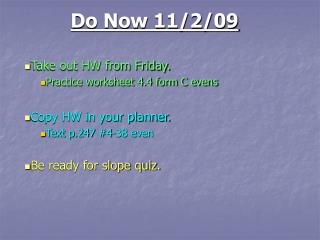DownloadDownload PresentationDo Now 11/2/09

# Do Now 11/2/09

Télécharger la présentation## Do Now 11/2/09

- - - - - - - - - - - - - - - - - - - - - - - - - - - E N D - - - - - - - - - - - - - - - - - - - - - - - - - - -
##### Presentation Transcript

1. Do Now 11/2/09 • Take out HW from Friday. • Practice worksheet 4.4 form C evens • Copy HW in your planner. • Text p.247 #4-38 even • Be ready for slope quiz.

2. 2) undefined 4) zero 6) positive 8) -11/6 10) 4/3 12) 0 14) -2/3 16) 3/5 18) undefined 20) 4/7 22) 0 24) 1/6 26) -7 28) -1 30) -4 32) a) From 1996 to 1998. b) From 1996 to 1998. c) Overall, the slope of both lines is positive meaning that fuel consumption has increased over the 10 year period. Homework Practice worksheet 4.4 Form C

3. Objective • SWBAT graph linear equations using slope-intercept form

4. Slope Review The slope m of a line passing through two points and is the ratio of the rise change to the run. y run rise x

5. Section 4.5 “Graph Using Slope-Intercept Form” SLOPE-INTERCEPT FORM- a linear equation written in the form y-coordinate x-coordinate y = mx + b slope y-intercept

6. y = 3x + 4 y = -3x + 2 y = 2 – 3x 3x +y = 2 The line has a slope of – 3 and a y-intercept of 2. The equation is in the formy =m x +b. So, the slope of the line is 3, and they-intercept is4. Rewrite the equation in slope-intercept form by solving for y. Identifying Slope and the Y-Intercept Slope of 3 means: y = mx + b Is this in slope-intercept form?

7. 3x –12 =3y y y x – 4 3x – 12 3x – 3y = 12 ANSWER The line has a slope of 1 and a y-intercept of –4. = 3 = Identify the slope and y-intercept of the line with the given equation. Rewrite the equation in slope-intercept form by solving for y. Rewrite original equation. Divide 3 by equation. Simplify.

8. Graph an Equation Using the Slope-Intercept Form Graph the equation y = 2 - 2x. y-axis 5 4 Rewrite in slope-intercept form 3 2 1 slope y-intercept -6 -5 -4 -3 -2 -1 0 1 2 3 4 5 6 x-axis -1 Slope of -2 means: -2 OR -3 Slope of -2 means: -4 -5

9. Graph an Equation Using the Slope-Intercept Form Graph the equation y = -3 + 2/3x. y-axis 5 4 Rewrite in slope-intercept form 3 2 1 slope y-intercept -6 -5 -4 -3 -2 -1 0 1 2 3 4 5 6 x-axis -1 Slope of 2/3 means: -2 OR -3 Slope of 2/3 means: -4 -5

10. = 3 = – 2 m b and – 2x + 3 y = Graph Using Slope and the Y-Intercept Graph the equation2x +y = 3. STEP1 Slope of -2 means: Rewrite the equation in slope-intercept form. STEP2 Identify the slope and the y-intercept. STEP3 Plot the point that corresponds to the y-intercept,(0, 3). STEP4 Use the slope to locate a second point on the line. Draw a line through the two points.

11. 2 x 3 = 1 = 2/3 b m and y = + 1 Graph Using Slope and the Y-Intercept Graph the equation3y – 2x = 3. Slope of 2/3 means: STEP1 Rewrite the equation in slope-intercept form. STEP2 Identify the slope and the y-intercept. STEP3 Plot the point that corresponds to the y-intercept,(0, 1). STEP4 Use the slope to locate a second point on the line. Draw a line through the two points.

12. Parallel Lines two lines in the same plane are parallel if they never intersect. Because slope gives the rate at which a line rises or falls, two lines with the SAME SLOPE are PARALLEL.

13. – 2 2 – 1 – 2 1 = = – 6 – 3 5 3 – 5 – 1 –0 1 – 5 – (–3) – 1 –2 = = 3 = – 3 – (–1) 0 –5 – 2 – 4 = Line aand line chave the same slope, so they are parallel. Determine which of the lines are parallel. Find the slope of each line. Line a:m= Line b: m= Line c: m=

14. HomeworkPractice Form C evens • 2) slope = -6; y-int = 19 • 4) slope = -3/2; y-int = 4 • 6) slope = 4/3; y-int = 3 • 8) slope = 4/9; y-int = -2 • 10 – 18) on overhead • 20) line through (-1,3) and (0,-3) and line through (0,2) and (1,-4) both have slopes of -6 • 22) parallel; slopes of 0.5 • 24) -38 • 26) -2 • 28) \$6; the cost increases by \$2 per hour

15. 2) slope -4; y-int 10 4) slope -4; y-int 3 6) slope -2/5; y-int 2 8) slope -3/2; y-int ½ 10) slope -1; y-int 3/5 12) slope undefined; y-int none 14) B 16) overhead 18) overhead 20) overhead 22) line through (-1,-4) and (0,2) and line through (1,3) and (2,9) 24) no 26) no 28) yes 30) a&b) overhead c) \$12 Practice Form B#2-22 even